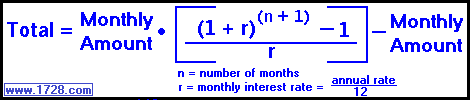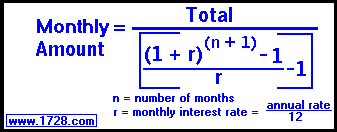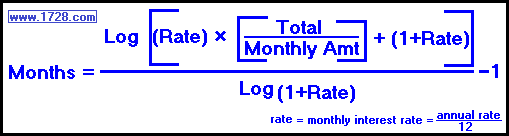Calculating a Monthly Annuity

 1) Solving the Total AmountYou set up an annuity in which you will pay \$150 per month for 20 years at 7 per cent annual interest. What is the total amount this will yield in 20 years? Using the formula above, the easiest amount to find is the monthly amount of \$150. For the interest rate 'r', we have to convert it from annual to monthly. .07 ÷ 12 = 0.0058333333 per month. Since this is a monthly annuity, we have to change the time from years to months. 20 years = 240 months. Now we put these amounts into the formula: Total = 150 • ([1.0058333333241 -1] ÷ .00375) - 150 Total = 150 • ([4.0622981589 -1] ÷ .00375) -150 Total = 150 • (3.0622981589 ÷ .00375) -150 Total = 150 • (524.9653986745) -150 Total = 78,594.81

 2) Solving the Monthly AmountYou set up a pension plan with an annual interst rate of 8 per cent, for 35 years and you would like this to result in \$1,000,000.00 for your retirement. How much must you invest each month? In this case, the easiest amount to find is the total which is \$1,000,000. As in the previous example, we need to convert the interst rate to a monthly rate and convert the years into months. 8% per year = .08/12 = 0.0066666666 per month and 35 years = 420 months. Entering the amounts into the above formula we have: Monthly Amount = 1,000,000 ÷ [(1.0066666666421 -1) ÷ (.0066666666) -1] Monthly Amount=1,000,000 ÷ [16.4011668971 -1 ÷ (.0066666666) -1] Monthly Amount=1,000,000 ÷ [15.4011668971 ÷ (.0066666666) -1] Monthly Amount = 1,000,000 ÷ (2,310.1750345608 -1); Monthly Amount = 1,000,000 ÷ (2,309.1750345608); Monthly Amount = 433.0550889531 Monthly Amount = 433.06

 3) Solving for the MonthsYou decide to invest \$250 per month in a 7.5% annual interest rate pension plan and you'd like to retire with \$500,000. How long will this take? For this calculation. we need to use the monthly rate which is .075/12 = .00625. First, we'll enter these amounts: (.00625) × (500,000 / 250) + (1.00625) = 13.50625 Taking the logarithm of this and dividing it by the logarithm of the denominator: Log (13.50625) ÷ Log(1.00625) = 1.1305347842 ÷ 0.0027058934 = 417.8046312859 Finally, the formula says we have to subtract 1 from this number: 416.8046312859 months It takes this number of months to yield \$500,000. Dividing this by 12 yields 34.7337192738 years.